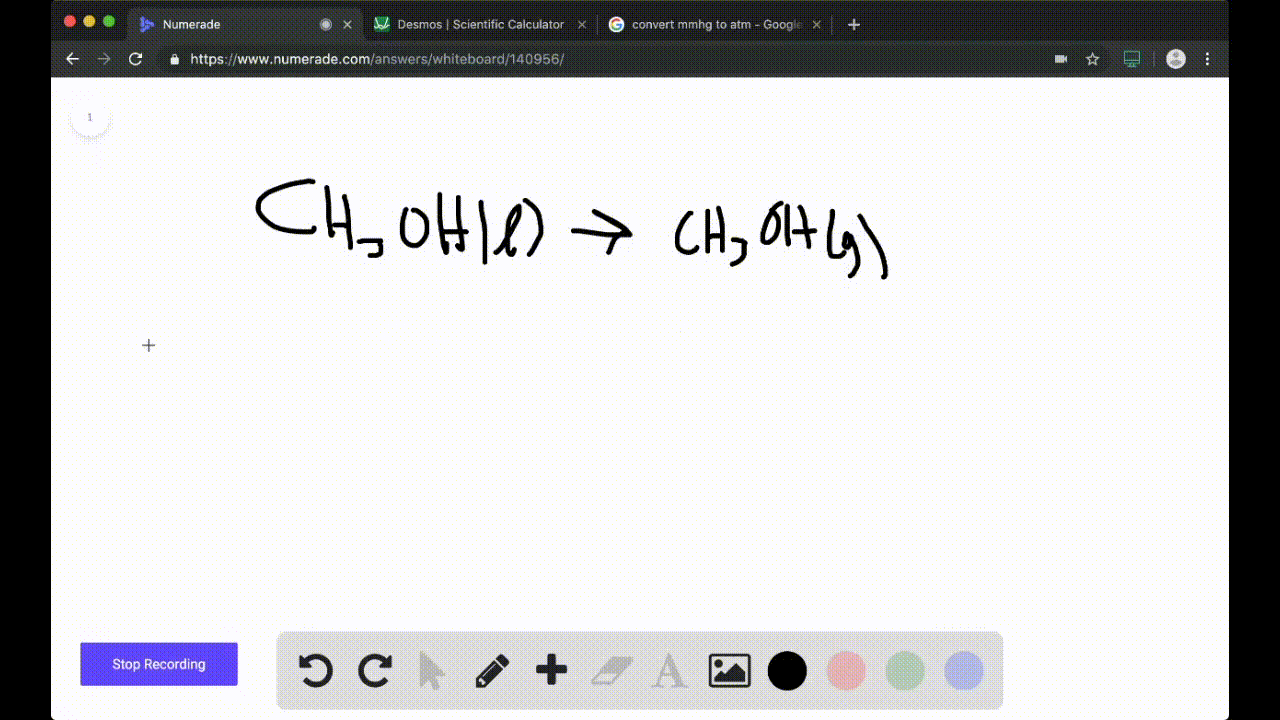### Problem 70

### Consider the evaporation of methanol at $25.0^{\c… 05:24### Our team of expert educators are currently working on this. #### Get notified when this problem is solved. Our educator team will work on creating an answer for you in the next 6 hours. Problem 69 Consider the sublimation of iodine at$25.0^{\circ} \mathrm{C} :$$$\mathrm{I}_{2}(s) \longrightarrow \mathrm{I}_{2}(g)$$ \begin{equation}\begin{array}{l}{\text { a. Find } \Delta G_{\mathrm{rxn}}^{\circ} \text { at } 25.0^{\circ} \mathrm{C} \text { . }} \\ {\text { b. Find } \Delta G_{\mathrm{rm}} \text { at } 25.0^{\circ} \mathrm{C} \text { under the following nonstandard }} \\ {\text { conditions: }} \\ \quad{\text { i. } P_{1_{2}}=1.00 \mathrm{mm} \mathrm{Hg}} \\ \quad{\text { ii. } P_{1_{2}}=0.100 \mathrm{mm} \mathrm{Hg}} \\ {\text { c. Explain why iodine spontaneously sublimes in open air at }} \\ {25.0^{\circ} \mathrm{C} .}\end{array}\end{equation} Answer PART$\mathrm{A} : \Delta G^{\circ}=19.3 \mathrm{kJ}$PART$\mathrm{B}-\mathrm{I} : \Delta G=2.9 \mathrm{kJ}$PART B-II:$\Delta G=-2.9 \mathrm{kJ}\$
PART C: See explanation.

## Discussion

You must be signed in to discuss.

## Video Transcript

No transcript available